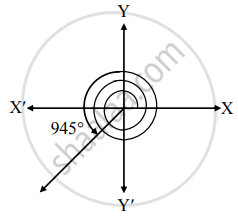# Draw the angle of the following measure and determine their quadrant. 945° - Mathematics and Statistics

Diagram
Sum

Draw the angle of the following measure and determine their quadrant.

945°

#### SolutionFrom the figure, the given angle terminates in quadrant III.

Concept: Angles in Standard Position
Is there an error in this question or solution?

#### APPEARS IN

Balbharati Mathematics and Statistics 1 (Arts and Science) 11th Standard Maharashtra State Board
Chapter 1 Angle and its Measurement
Exercise 1.1 | Q 1. (B) (v) | Page 8